## Quiz 3 : Cost BehaviorLooking for Accounting Homework Help?# Quiz 3 : Cost Behavior

1.Determine the cost of equation for total cost of labor: Given:Let number of classes taught and total cost of labor be x and y.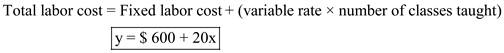2.Calculate the total variable labor cost: The total variable labor cost is calculated as follows:Hence, the total variable labor cost last month is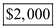3.Calculate total labor cost: Substitute 100 for number of classes taught in above determined total cost of labor equation.Hence, the total labor cost last month is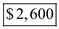4.Calculate unit labor cost: Divide total labor cost with number of classes taught.Hence, the unit cost of labor (per class) for last month is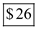5. Calculate total labor cost when number of classes are increased by 50%: If C increases the number of classes by 50%, the number of classes would becomeSubstitute 150 for number of classes taught in above determined total cost of labor equation.Calculate revised unit labor cost: The unit labor cost is calculated as follows:The unit labor cost went down because the fixed cost is spread over a greater number of classes taught.

Cost behavior is the term used to express the responsiveness of cost with respect to other factor(s). A manager should be able to understand and assess the changes in costs that result from the change of activity. In this regard, the knowledge of cost behavior is very much needed. Suppose, if there is an excess capacity it minimally cover variable costs that may be totally appropriate. Knowing what costs are variable and what costs are fixed can help a manager make better bid.

Corazon manufacturing company purchasing department has five agents. Given :The activity rate is calculated as:Activity rate is \$7 per purchase order. 2.(a)The total activity availability is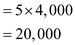The total activity availability is 20,000 purchase orders. (b)Unused capacity is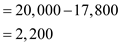3.(a)The total activity availability is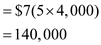The total activity availability is 140,000 purchase orders. (b)Unused capacity is4.The total activity availability is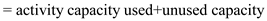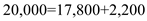5.One purchasing agent working half time could process 18,000 purchase orders (4.5 × 4,000). Since 17,800 purchase orders are processed, the unused capacity would be 200 purchase orders (18,000 - 17,800).